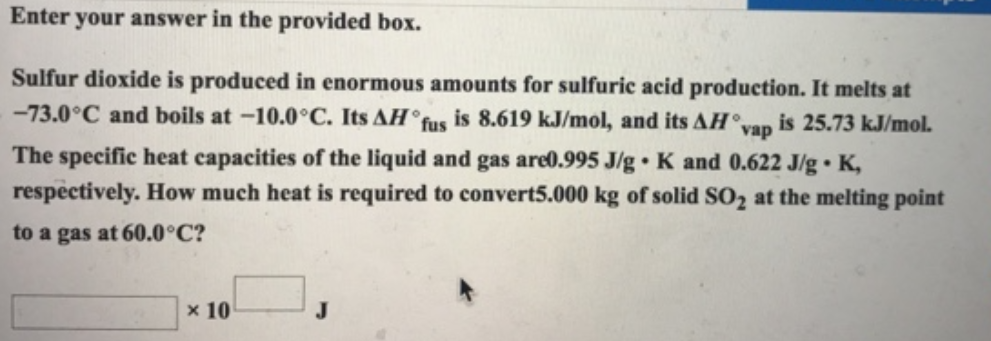# Problem: Enter your answer in the provided box. Sulfur dioxide is produced in enormous amounts for sulfuric acid production. It melts at 73.0 °C and boils at -10.0 °C. Its  ΔH°fus is 8.619 kJ/mol, and its ΔH°vap is 25.73 kJ/mol. The specific heat capacities of the liquid and gas are 0.995 J/g. K and 0.622 J/g. K, respectively. How much heat is required to convert 5.000 kg of solid SO2 at the melting point to a gas at 60.0 °C?

🤓 Based on our data, we think this question is relevant for Professor Islam's class at UIC.

###### FREE Expert Solution###### Problem Details

Sulfur dioxide is produced in enormous amounts for sulfuric acid production. It melts at 73.0 °C and boils at -10.0 °C. Its  ΔH°fus is 8.619 kJ/mol, and its ΔH°vap is 25.73 kJ/mol. The specific heat capacities of the liquid and gas are 0.995 J/g. K and 0.622 J/g. K, respectively. How much heat is required to convert 5.000 kg of solid SO2 at the melting point to a gas at 60.0 °C?